Next: Euler's formula: Up: Special Numbers and Functions Previous: Special Numbers and Functions

# How to compute digits of ?

Symbolic Computation software such as Maple or Mathematica can compute 10,000 digits of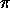in a blink, and another 20,000-1,000,000 digits overnight (range depends on hardware platform).

It is possible to retrieve 1.25+ million digits ofvia anonymous ftp from the site wuarchive.wustl.edu, in the files pi.doc.Z and pi.dat.Z which reside in subdirectory doc/misc/pi. New York's Chudnovsky brothers have computed 2 billion digits ofon a homebrew computer.

The current record is held by Yasumasa Kanada and Daisuke Takahashi from the University of Tokyo with 51 billion digits of(51,539,600,000 decimal digits to be precise).

Nick Johnson-Hill has an interesting page of trivia at: http://www.users.globalnet.co.uk/ nickjh/Pi.htm

The new record for the number of digits ofis 4.29496 billion decimal digits of pi were calculated and verified by 28th August '95.

Related documents are available with anonymous ftp to www.cc.u-tokyo.ac.jp.

` ftp://www.cc.u-tokyo.ac.jp/`

This computations were made by Yasumasa Kanada, at the University of Tokyo.

There are essentially 3 different methods to calculateto many decimals.

1. One of the oldest is to use the power series expansion of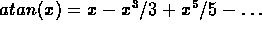together with formulas like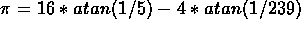. This gives about 1.4 decimals per term.
2. A second is to use formulas coming from Arithmetic-Geometric mean computations. A beautiful compendium of such formulas is given in the bookand the AGM, (see references). They have the advantage of converging quadratically, i.e. you double the number of decimals per iteration. For instance, to obtain 1 000 000 decimals, around 20 iterations are sufficient. The disadvantage is that you need FFT type multiplication to get a reasonable speed, and this is not so easy to program.
3. A third one comes from the theory of complex multiplication of elliptic curves, and was discovered by S. Ramanujan. This gives a number of beautiful formulas, but the most useful was missed by Ramanujan and discovered by the Chudnovsky's. It is the following (slightly modified for ease of programming):

Set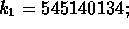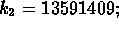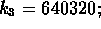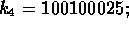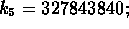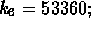Then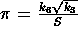, where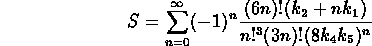The great advantages of this formula are that

1) It converges linearly, but very fast (more than 14 decimal digits per term).

2) The way it is written, all operations to compute S can be programmed very simply. This is why the constant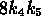appearing in the denominator has been written this way instead of 262537412640768000. This is how the Chudnovsky's have computed several billion decimals.

An interesting new method was recently proposed by David Bailey, Peter Borwein and Simon Plouffe. It can compute the Nth hexadecimal digit of Pi efficiently without the previous N-1 digits. The method is based on the formula: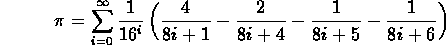in O(N) time and O(log N) space. (See references.)

The following 160 character C program, written by Dik T. Winter at CWI, computesto 800 decimal digits.

```     int a=10000,b,c=2800,d,e,f,g;main(){for(;b-c;)f[b++]=a/5;
for(;d=0,g=c*2;c-=14,printf("%.4d",e+d/a),e=d%a)for(b=c;d+=f[b]*a,
f[b]=d%--g,d/=g--,--b;d*=b);}```

References

P. B. Borwein, and D. H. Bailey. Ramanujan, Modular Equations, and Approximations toAmerican Mathematical Monthly, vol. 96, no. 3 (March 1989), p. 201-220.

D. H. Bailey, P. B. Borwein, and S. Plouffe. A New Formula for Picking off Pieces of Pi, Science News, v 148, p 279 (Oct 28, 1995). also at ` http://www.cecm.sfu.ca/~pborwein `

J.M. Borwein and P.B. Borwein. The arithmetic-geometric mean and fast computation of elementary functions. SIAM Review, Vol. 26, 1984, pp. 351-366.

J.M. Borwein and P.B. Borwein. More quadratically converging algorithms for. Mathematics of Computation, Vol. 46, 1986, pp. 247-253.

Shlomo Breuer and Gideon Zwas Mathematical-educational aspects of the computation ofInt. J. Math. Educ. Sci. Technol., Vol. 15, No. 2, 1984, pp. 231-244.

David Chudnovsky and Gregory Chudnovsky. The computation of classical constants. Columbia University, Proc. Natl. Acad. Sci. USA, Vol. 86, 1989.

Classical Constants and Functions: Computations and Continued Fraction Expansions D.V.Chudnovsky, G.V.Chudnovsky, H.Cohn, M.B.Nathanson, eds. Number Theory, New York Seminar 1989-1990.

Y. Kanada and Y. Tamura. Calculation ofto 10,013,395 decimal places based on the Gauss-Legendre algorithm and Gauss arctangent relation. Computer Centre, University of Tokyo, 1983.

Morris Newman and Daniel Shanks. On a sequence arising in series for. Mathematics of computation, Vol. 42, No. 165, Jan 1984, pp. 199-217.

E. Salamin. Computation ofusing arithmetic-geometric mean. Mathematics of Computation, Vol. 30, 1976, pp. 565-570

David Singmaster. The legal values of. The Mathematical Intelligencer, Vol. 7, No. 2, 1985.

Stan Wagon. Isnormal? The Mathematical Intelligencer, Vol. 7, No. 3, 1985.

A history of. P. Beckman. Golem Press, CO, 1971 (fourth edition 1977)and the AGM - a study in analytic number theory and computational complexity. J.M. Borwein and P.B. Borwein. Wiley, New York, 1987.Next: Euler's formula: Up: Special Numbers and Functions Previous: Special Numbers and Functions

Alex Lopez-Ortiz
Mon Feb 23 16:26:48 EST 1998